Open Access ArticleThis Open Access Article is licensed under a
Creative Commons Attribution 3.0 Unported Licence

# Electrochemistry of actinides on reduced graphene oxide: craving for the simultaneous voltammetric determination of uranium and plutonium in nuclear fuel

Saurav K. Guin *, Arvind S. Ambolikar and J. V. Kamat
Fuel Chemistry Division, Bhabha Atomic Research Centre, Trombay, Mumbai-400 085, India. E-mail: sauravkrguin@yahoo.co.in; skguin@barc.gov.in; Fax: +91-22-2550-5151; Tel: +91-22-2559-4598

Received 26th May 2015 , Accepted 1st July 2015

First published on 2nd July 2015

## Abstract

The aqueous electrochemistry of plutonium (Pu) has been explored for the first time on the reduced graphene oxide modified glassy carbon electrode (rGO/GC). It has been confirmed that rGO/GC can catalyse the Pu(IV)/Pu(III) redox reaction in 1 M H2SO4 and that finally leads to the high analytical sensitivity of anodic square wave voltammetric determination of Pu. However, the sensitivity of rGO/GC decreases in the actual nuclear fuel sample [i.e. Mark-I (U, Pu)C fuel dissolved in 1 M H2SO4] due to the interference of uranium (U). Furthermore, the cathodic square wave voltammograms of U(VI) in the sample solution have been found to be very inconsistent. That inconsistency is explained by the interfacial coupled chemical reaction between U(IV) (just produced at the working electrode) and Pu(IV) diffusing from the bulk of the solution to the vicinity of the working electrode. That is why the quantitative determination of uranium in the presence of plutonium is not feasible by simple voltammetric techniques on any working electrode. Although rGO/GC shows good analytical robustness, reproducibility, repeatability, fast analysis and least requirement of additional reagents; but challenge still exists in the analytical merits for the determination of Pu in nuclear fuel sample in competition with the biamperometric method.

## 1. Introduction

Electricity or power is one of the most critical components of infrastructure affecting the economic growth and welfare of India. India's three-stage atomic energy programme has been planned for a comprehensive mission-oriented long-term sustainable generation of electricity. In the first stage, India has reached to a total nuclear plant capacity of 5780 MW of electricity by safely operating eighteen pressurised heavy water reactors, two boiling water reactors and one pressurised water reactor.1 The Fast Breeder Test Reactor (FBTR) built at Indira Gandhi Centre for Atomic Research, Kalpakkam, is a fore-runner in the second stage of the Indian nuclear power programme. The reactor was made critical with a small core containing hyper-stoichiometric mixed plutonium (Pu)–uranium (U) carbide (Pu0.7U0.3)C (Mark-I) as a driver fuel.2 On this platform, precise and accurate analytical techniques are essential to control the desired quality of the nuclear fuel as well as to account the nuclear materials needed for non-proliferation and safety purposes.

The uranium and plutonium in a nuclear fuel sample can be simultaneously determined by α-spectrometry,3 spectrophotometry,4–7 thermal ionization mass spectrometry,8 neutron activation analysis,9 potentiometry10etc. Redox titration methods, employing a visual indicator or electrometric end point detection, are widely used for the determination of uranium and plutonium independently in the uranium–plutonium mixed oxides.11–19 The major issue in the quantitative redox titration of a mixed uranium–plutonium solution is the interfering redox reaction between U(IV) and Pu(IV) in acidic solutions. Following discussed redox titrimetric methodologies have been strategically developed to separately determine U and Pu avoiding their mutual interference. Plutonium is determined by the redox titration method developed by J. L. Drummond and R. A. Grant. In this method, Pu(III) and Pu(IV) are oxidized to Pu(VI) by excess argentic oxide (AgO) in 1 M H2SO4 solution followed by the destruction of excess AgO by sulphamic acid. Then Pu(VI) is quantitatively reduced to Pu(IV) by known excess of standard Fe(II), which is titrated with standard potassium dichromate (K2Cr2O7) solution. If U is present in the solution then it exists as U(VI) and thus it does not interfere in the determination of Pu(IV).19 Uranium is determined separately by the Davies and Gray method,18 modified by the New Brunswick Laboratory.11 In this method, the U(VI) solution (in 1 M H2SO4 + 12 M H3PO4) is quantitatively reduced to U(IV) by excess Fe(II), which is selectively oxidized by the addition of a solution containing sulphamic acid, ammonium molybdate and nitric acid. Then the reaction mixture is diluted by 1 M H2SO4 (containing catalytic amount of V(IV)) down to 3 M H3PO4 concentration. Under this condition, U(IV) is oxidized to U(VI) by reducing Fe(III) to Fe(II), which is titrated by standard K2Cr2O7 solution. If the solution contains Pu, it does not interfere in the end point result, because whatever Pu(IV, VI) is reduced to Pu(III) by Fe(II) in the reduction step; the same amount of Pu(III) is back oxidized by Mo(VI) in the oxidation step to Pu(IV). However, the recovery of Pu from the highly complexing H3PO4 medium is cumbersome. Therefore, a couple of alternative redox titrimetric methods have been developed in our laboratory for biamperometric determination of U and Pu.12,14,16 However, those redox titrimetric methods mostly require separate exercise and reagents for the determination of U and Pu in the same sample.

Controlled potential coulometry is another well established and routinely employed technique for precise and accurate determination of uranium and plutonium.20–26 The quantitative conversion of U(VI) to U(IV) in 1 M H2SO4 is carried out at −0.325 V versus saturated calomel electrode (SCE) on mercury pool electrode for the determination of uranium. Similarly, the Pu(III) is quantitatively oxidized to Pu(IV) in H2SO4 at 0.7 V versus SCE on platinum wire gauge electrode for the determination of plutonium. Therefore, two different working electrodes are necessary for the coulometric determination of U and Pu. A couple of initiatives have been taken to replace two separate working electrodes by single working electrode (like graphite electrode etc.) to simultaneously determine U and Pu in the same sample.27–29 However, the chance of interference from the redox reaction between Pu(IV) and U(IV) still exists during the coulometric determination of U in the presence of Pu on a single working electrode. Therefore, a pre-reduction step at 0.085 V vs. SCE is performed to completely reduce all Pu into Pu(III) and then the potential is set at −0.325 V vs. SCE for the reduction of U(VI) to U(IV). Although coulometry is an absolute electroanalytical method, but the total duration of analysis for a sample is effectively long.

In contrast, voltammetry is one of the rapid electroanalytical techniques capable for fast, precise and accurate quantification of the analytes.30 Therefore; it was of interest to develop easier and rapid voltammetric methodology for the determination of uranium and plutonium in the nuclear fuels. The graphene materials have been emerging in the electrocatalysis and electroanalysis as the potent surface modifying agents for the working electrodes, where the graphene materials can selectively increase the rate of heterogeneous electron transfer as well as the selectivity of the determination of the analyte. Authors have recently explored the electrocatalytic action of electrochemically reduced graphene oxide (rGO) modified glassy carbon electrode (i.e. rGO/GC) in the electrochemistry of [UVIO2(CO3)3]4−/[UVO2(CO3)3]5− redox couple.31 There it was evidenced that in situ generated topological defects introduce significant in-plane distortions and strains in the surrounding lattice of rGO resulting into its higher reactivity towards the electrochemistry of U. However, the aqueous electrochemistry of plutonium on graphene material is yet unexplored. Therefore, the prime focus of this article is to study the electrochemistry of Pu(IV)/P(III) redox couple in 1 M H2SO4 on rGO/GC. In addition to this it was found in the literature that single-walled carbon nanotube modified gold electrode, without addressing any interference from the interfacial redox chemistry of U(IV) and Pu(IV), apparently showed the ability to precisely, accurately and simultaneously determine U and Pu down to μM (or ng g−1 to μg g−1) concentration ranges!32 Thus we have also explored the validity of the simple voltammetric techniques on the solid electrodes for the simultaneous quantitative determination of uranium and plutonium in FBTR fuel. This paper evidently reports that the interference from the interfacial redox chemistry of U(IV) and Pu(IV) restricts the quantitative determination of uranium in the presence of plutonium on any solid electrodes (irrespective of the type of surface modifications) by simple voltammetric methods such as cyclic voltammetry, differential pulse voltammetry and square wave voltammetry.

## 2. Experimental

Caution! 239Pu is an α-active nuclide with a half-life of 2.4 × 104 years. This corresponds to the production of 138 × 106 α-particles min−1 mg−1 of Pu. This radioactive material must be handled carefully in a radiological facility with appropriate equipment to avoid any health risk caused by the radiation exposure. Therefore, all the experiments were performed with proper training and great care in a suitable place under safe conditions.33

Guaranteed reagent (G.R.) grade sulphuric acid (H2SO4), extra pure ACS grade uranyl nitrate hexahydrate [UO2(NO3)2·6H2O] were used as received. All the solutions were prepared using ultra pure water (Milli-Q, Millipore, 18.2 MΩ cm). The GO purchased from M/s. Global Nanotech were used as received. The product certificate (received from M/s. Global Nanotech) is available elsewhere.31 The GC electrode was modified with GO by drop casting about 0.16 μg (optimized to have least amount of capacitive current) GO dispersed in nanopure water followed by gentle drying under IR lamp. The GO modified GC electrode was electrochemically reduced by scanning the potential window from 0 V to −1.6 V (vs. Ag/AgCl(saturated KCl) reference electrode) at a scan rate of 50 mV s−1 for only one complete cycle in saturated Na2CO3 solution (pH ∼ 12.3) as discussed elsewhere.31

A stock solution of 7.618 mg g−1 (or 31.87 mM) of standard plutonium was prepared from the solid working reference, potassium plutonium sulphate dihydrate (K4Pu(SO4)4·2H2O); which was prepared in our laboratory by a method as described elsewhere.34 In brief, a mixture of K2SO4 and Pu(SO4)2 in a molar ratio of 2:1 was slowly evaporated in 1 M H2SO4 to prepare crystals of (K4Pu(SO4)4·2H2O). The crystals were washed several times with absolute alcohol followed by drying in a current of air. Then the anhydrous K4Pu(SO4)4 was prepared by heating the hydrated crystals at 340 °C for about three hours until constant weight. A known amount of the anhydrous standard K4Pu(SO4)4 was quantitatively dissolved in 15 mL of 3 M HNO3 and the solution was fumed with 1 M H2SO4 to convert into sulfate form. The residue was again treated with 1 M H2SO4 and evaporated to dryness. This particular step was repeated several times to ensure the complete removal of nitrate. Finally, the stock solution of 7.618 mg g−1 (or 31.87 mM) plutonium in 1 M H2SO4 was prepared by quantitatively diluting the residue with 1 M H2SO4.

FBTR Mark-I fuel sample solution was prepared by following the method published elsewhere.35 The fuel samples were analysed by biamperometric method with the sample solution having plutonium concentration in the range of 2–6 mg g−1. The reported values (by biamperometry) of U and Pu in this sample are 1.575 mg g−1 and 3.799 mg g−1, respectively. For any conversion in the concentration units, we have used 1 mM of Pu(IV) and U(VI) equivalent to 0.239 mg g−1 of Pu(IV) and 0.238 mg g−1 of U(VI), respectively.

The electrochemical experiments were performed at room temperature (T = 298 K) in a conventional three-electrode cell by using CHI-450B electrochemical workstation. There was no uncompensated resistance in the voltammetric results. A commercial glassy carbon (GC) (φ = 3 mm) or modified GC electrode was used as the working electrode, Ag/AgCl/KCl (saturated) (EAg/AgCl = +0.197 V vs. standard hydrogen electrode) was used as the reference electrode and a platinum wire acted as the counter electrode. All the potentials quoted are with respect to the Ag/AgCl(saturated KCl) reference electrode. Prior to perform the electrochemical experiments, the working solution was purged with high purity nitrogen for 15 min to remove the dissolved oxygen from the solution. The GC electrode was polished by using alumina slurries with different powders of size down to 0.05 μm. After each polishing, the electrode was thoroughly rinsed with water.

## 3. Results and discussion

Fig. 1 shows the cyclic voltammogram of 10 mM Pu(IV) in 1 M H2SO4 solution on (i) GC and (ii) rGO/GC electrodes at a scan rate of 10 mV s−1. The cathodic (Ecp) and anodic (Eap) peak potentials for Pu(IV)/Pu(III) redox couple are appeared at 0.092 V and 0.666 V, respectively, on GC (Fig. 1(i)). The separation between the peak potentials (ΔEp) is calculated as 0.574 V. The cathodic peak is very broad; whereas the anodic peak is comparatively sharper. However, for the same reaction on rGO/GC, Ecp is shifted to lesser cathodic potential (0.398 V) and Eap is shifted to lesser anodic potential (0.605 V) leading to a narrower peak separation (0.207 V) (Fig. 1(ii)). Therefore, the overpotentials for both the cathodic and anodic reactions are significantly decreased indicating that rGO can catalyse the electrochemistry of Pu(IV)/Pu(III) redox couple in 1 M H2SO4.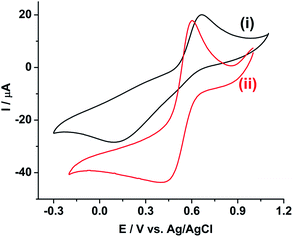Fig. 1 Cyclic voltammograms of 10 mM Pu(IV) in 1 M H2SO4 solution on (i) GC and (ii) rGO/GC electrodes at a scan rate of 10 mV s−1.

Fig. 2a shows the effect of the scan rates (υ) on the cyclic voltammograms of 10 mM Pu(IV) in 1 M H2SO4 solution on GC electrode. The flux of the analyte towards and outward the electrode increases with increasing the scan rate. Therefore, the current of the voltammograms systematically increases with increasing the scan rate. It is known that the peak current is proportional to the square root of scan rate for diffusion controlled electron transfer reaction, whereas, the peak current proportionally varies with the scan rate for adsorption controlled electron transfer reaction. Therefore, the theoretical slopes of ln(−Ip) vs. ln(υ) plot should be 0.5 and 1 for diffusion controlled and adsorption controlled electron transfer reactions, respectively. The inset of Fig. 2a shows the linear variation of ln(−Ip) as a function of ln(υ) with a slope of 0.37 (R2 = 0.997) and 0.38 (R2 = 0.993) for (1) cathodic and (2) anodic peak currents, respectively. Therefore, it can be inferred that the redox reaction of Pu(IV)/Pu(III) couple on GC is governed only by the diffusion controlled mechanism. The other electrochemical data such as Ecp, Eap, ΔEp, formal potential of the redox couple (E0); half-cathodic peak potential (Ecp/2); half-anodic peak potential (Eap/2); effective charge transfer coefficient for the cathodic reaction (n*αc) and effective charge transfer coefficient for the anodic reaction (n*αa) are listed in Table 1. Here, n* and αc, αa designate the electron stoichiometry in the rate determining step and charge transfer coefficient for cathodic and anodic reactions, respectively. For this well characterized reaction, n* can be considered as equal to the stoichiometric number of electrons i.e., n = n* = 1. The E0, n*αc and n*αc are calculated at each scan rate by eqn (1–3):36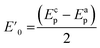(1)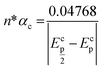(2)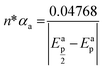(3)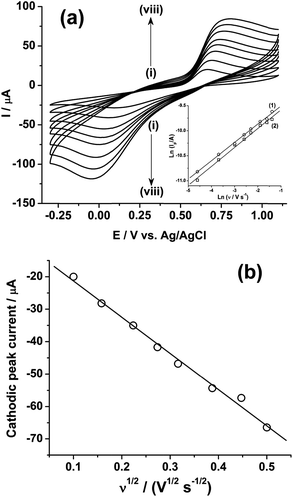Fig. 2 (a) Cyclic voltammograms of 10 mM Pu(IV) in 1 M H2SO4 solution on GC electrode at a scan rate (υ) of (i) 10, (ii) 25, (iii) 50, (iv) 75, (v) 100, (vi) 150, (vii) 200 and (viii) 250 mV s−1. The inset shows the plots of ln(Ip) versus ln(υ) for (1) cathodic and (2) anodic peaks. (b) Plot of the cathodic peak current versus the square root of the scan rate (υ1/2).
Table 1 Electrochemical parameters of the cyclic voltammograms of 10 mM Pu(IV) in 1 M H2SO4 solution on GC electrode at different scan rates (υ)
υ/V s−1 E cp/V E cp/2/V E ap/V E ap/2/V ΔEp/V E0/V n*αc n*αa
0.010 0.092 0.337 0.666 0.587 0.574 0.379 0.19 0.60
0.025 0.058 0.281 0.691 0.607 0.633 0.374 0.21 0.57
0.050 0.033 0.239 0.714 0.618 0.681 0.373 0.23 0.50
0.075 0.012 0.218 0.729 0.625 0.717 0.370 0.23 0.46
0.100 0.001 0.201 0.743 0.631 0.742 0.372 0.24 0.43
0.150 −0.022 0.174 0.769 0.640 0.791 0.373 0.24 0.37
0.200 −0.035 0.149 0.788 0.649 0.823 0.377 0.26 0.34
0.250 −0.045 0.142 0.807 0.656 0.852 0.381 0.25 0.32

It can be seen from Table 1 that, the values of E0 (0.375 ± 0.004 V vs. Ag/AgCl); n*αc (0.23 ± 0.02) and n*αa (0.45 ± 0.10) are almost independent of the scan rate; although ΔEp is increased from 0.574 V at υ = 0.010 V s−1 to 0.852 V at υ = 0.250 V s−1. This suggests that the electrochemistry of Pu(IV)/Pu(III) redox couple on GC electrode is quasi-reversible in nature. Since, Pu(IV) is more stable in 1 M H2SO4 compared to Pu(III). Therefore, the small value of αc (αc = 0.23; considering n* = 1) is responsible for the broad cathodic peak of the CV. It should be noted that in an old solution, a certain fraction of plutonium also exists in Pu(VI) state and its reduction to Pu(III) via Pu(IV) also broadens the cathodic peak.

Fig. 2b shows the linear variation of Icp with the square root of the scan rate (υ1/2). Therefore, the diffusion coefficient (D in cm2 s−1) of Pu(IV) in 1 M H2SO4 solution could be calculated as 1.2 × 10−6 cm2 s−1 by eqn (4):36–41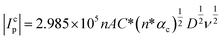(4)
where, n, A and C* are the stoichiometric number of electron (1 in present case), area of the electrode (0.071 cm2) and bulk concentration of Pu(IV). If we assume, the diffusion coefficients of Pu(IV) and Pu(III) are equal; then we can define the kinetic parameter (Ψ) as eqn (5):37–42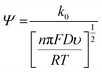(5)
where,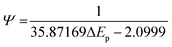(6)
and k0 is the standard rate constant of the reduction of Pu(IV). Therefore, k0 of the reduction of Pu(IV) on GC is calculated as 4.7 × 10−5 cm s−1 from the slope of the plot of [nπFDυ/(RT)]−1/2versus Ψ. The reversibility factor (Λ) of the redox reaction is defined as eqn (7):36,43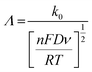(7)
The values of Λ for the redox reaction of Pu(IV)/Pu(III) couple on GC electrode lies between 6.94 × 10−2 (for υ = 0.010 V s−1) and 1.39 × 10−2 (for υ = 0.250 V s−1) representing the quasi-reversible electrochemistry of Pu(IV)/Pu(III) on GC electrode.

Fig. 3 shows the effect of the scan rates on the cyclic voltammograms of 10 mM Pu(IV) in 1 M H2SO4 solution on rGO/GC electrode. The current of the voltammograms is systematically increased with increasing the scan rate. The inset of Fig. 3a shows the linear variation of ln(−Ip) as a function of ln(υ) with a slope of 0.34 and 0.33 for (1) cathodic and (2) anodic peak currents, respectively. Therefore, the redox reaction of Pu(IV)/Pu(III) couple on rGO/GC is also governed by the diffusion controlled mass transfer mechanism. Table 2 shows the values of Ecp, Eap, ΔEp, E0, Ecp/2, Eap/2, n*αc, n*αa as a function of υ for the voltammograms of 10 mM Pu(IV) in 1 M H2SO4 solution on rGO/GC electrode. The values of E0 (0.465 ± 0.022 V vs. Ag/AgCl), n*αc (0.24 ± 0.05) and n*αa (0.59 ± 0.04) are almost independent of the scan rate; although ΔEp is increased from 0.207 V at υ = 0.010 V s−1 to 0.457 V at υ = 0.250 V s−1. This suggests that the electrochemistry of Pu(IV)/Pu(III) redox couple on rGO/GC electrode is also quasi-reversible in nature. No significant difference in the values of E0, n*αc and n*αc is observed for the electrochemistry of Pu(IV)/Pu(III) couple on rGO/GC compared to bare GC. However, the ΔEp becomes significantly narrower on rGO/GC compared to GC for all the scan rates. We have assumed that the D value of Pu(IV) should be independent of the nature of the electrode as long as the working solution is identical. Therefore, k0 of the reduction of Pu(IV) on rGO/GC is calculated as 1.9 × 10−4 cm s−1 from the slope of the plot of [nπFDυ/(RT)]−1/2versus Ψ considering the D of Pu(IV) as 1.2 × 10−6 cm2 s−1. Therefore, the standard electron transfer rate constant for the reduction of Pu(IV) is increased at rGO/GC compared to bare GC. This observation is in accordance with the results of the electrochemical reduction of [UVIO2(CO3)3]4− on rGO/GC electrode.31 The value of Λ for the redox reaction of Pu(IV)/Pu(III) couple on rGO/GC electrode lies between 2.8 × 10−1 (for υ = 0.010 V s−1) and 5.6 × 10−2 (for υ = 0.250 V s−1) representing the quasi-reversible electrochemistry of Pu(IV)/Pu(III) on rGO/GC electrode. The extent of the reversibility for the same redox couple increased on rGO/GC compared to bare GC. Therefore, the increase in the k0 value of the electron transfer reaction as well as the enhancement of the electrochemical reversibility at rGO/GC could be considered as the signature of electrocatalysis, which is mostly imposed by the in situ generated topological defects of rGO/GC.31,44,45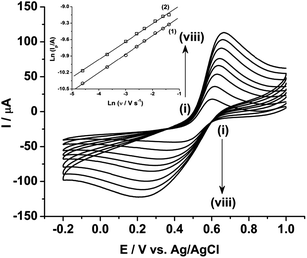Fig. 3 Cyclic voltammograms of 10 mM Pu(IV) in 1 M H2SO4 solution on rGO/GC electrode at a scan rate (υ) of (i) 10, (ii) 25, (iii) 50, (iv) 75, (v) 100, (vi) 150, (vii) 200 and (viii) 250 mV s−1. The inset shows the plots of ln(Ip) versus ln(υ) for (1) cathodic and (2) anodic peaks.
Table 2 Electrochemical parameters of the cyclic voltammograms of 10 mM Pu(IV) in 1 M H2SO4 solution on rGO/GC electrode at different scan rates (υ)
υ /V s−1 E cp/V E cp/2/V E ap/V E ap/2/V ΔEp/V E0/V n*αc n*αa
0.010 0.398 0.539 0.605 0.530 0.207 0.501 0.34 0.64
0.025 0.358 0.530 0.617 0.542 0.259 0.488 0.28 0.64
0.050 0.319 0.517 0.629 0.551 0.310 0.474 0.24 0.61
0.075 0.295 0.507 0.637 0.558 0.342 0.466 0.22 0.60
0.100 0.279 0.497 0.641 0.562 0.362 0.460 0.22 0.60
0.150 0.247 0.480 0.652 0.566 0.405 0.449 0.20 0.55
0.200 0.225 0.467 0.659 0.570 0.434 0.442 0.20 0.54
0.250 0.210 0.451 0.667 0.577 0.457 0.439 0.19 0.53

Fig. 4a shows the square wave voltammogram (SWV) of 10 mM Pu in 1 M H2SO4 in the cathodic scan direction. The GC electrode shows a broad cathodic SWV peak with the peak potential at 0.150 V and peak current of −5.77 μA (Fig. 4a(i)). The peak potential decreases to 0.564 V with increasing the peak current to −27.5 μA at rGO/GC electrode (Fig. 4a(ii)). As discussed in the earlier sections, due to the small value of the effective charge transfer coefficient for the cathodic reaction, the broad reduction peak is observed on both the GC and rGO/GC electrodes. Therefore, the selection of cathodic SWV is not expected to be suitable for the quantitative analysis of plutonium. On the other hand, the GC electrode shows a comparatively sharper SWV anodic peak with the peak potential at 0.611 V and peak current of 10.4 μA (Fig. 4b(i)). The peak potential is decreased to 0.547 V with increasing the peak current to 51.0 μA for the same reaction at rGO/GC electrode (Fig. 4b(ii)). The sharper oxidation peaks are attributed to the higher value of the effective charge transfer coefficient for the anodic reaction. Therefore, the quantitative analysis of plutonium samples could be done by anodic SWV and thus, henceforth we have restricted our studies to the anodic SWV (ASWV) of plutonium.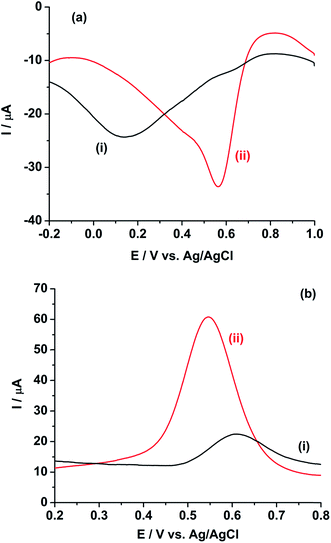Fig. 4 Square wave voltammograms of 10 mM Pu(IV) in 1 M H2SO4 solution on (i) GC and (ii) rGO/GC electrodes in the (a) cathodic and (b) anodic scan directions. Square wave amplitude = 25 mV; square wave frequency = 20 Hz; step potential increment = 1 mV; hold time at initial potential = 10 s.

Fig. 5 shows the ASWV of (i) 2.17, (ii) 2.86, (iii) 3.38 and (iv) 3.81 mg g−1 of Pu in 1 M H2SO4 on rGO/GC. The peak current (Ip) is linearly increased with increasing the concentration of Pu (inset of Fig. 5) with a sensitivity of 25.8 μA (mg g−1)−1. Fig. 6a shows ten repetitive ASWVs of the fuel sample on rGO/GC in the scan potential range 0.35 V to 0.75 V with Ip = 73.7 ± 0.06 μA.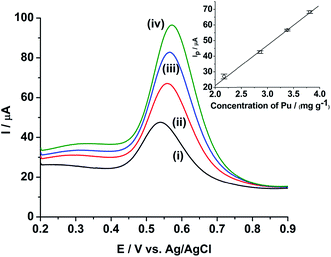Fig. 5 ASWVs of (i) 2.17, (ii) 2.86, (iii) 3.38 and (iv) 3.81 mg g−1 of Pu in 1 M H2SO4 on rGO/GC. Square wave amplitude = 25 mV; square wave frequency = 20 Hz; step potential increment = 1 mV; hold time at initial potential = 10 s. Inset shows the sensitivity plot for the same. The vertical bars shown in the inset represent the standard deviation (σ) of Ip obtained from ten replicate measurements for each concentration of Pu.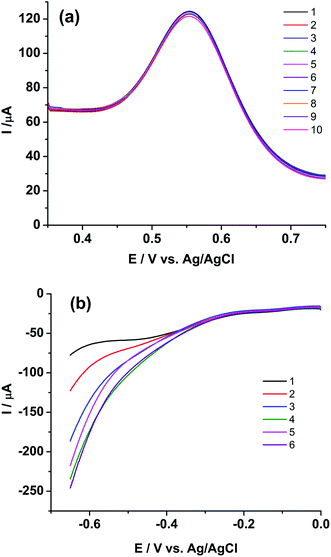Fig. 6 Square wave voltammograms of (U, Pu)C FBTR sample on rGO/GC for (a) anodic scan from 0.35 V to 0.75 V for analyzing Pu and (b) cathodic scan from 0 V to −0.65 V for analyzing U. Square wave amplitude = 25 mV; square wave frequency = 20 Hz; step potential increment = 1 mV; standby time at 0.35 V (for a) and at 0 V (for b) is 10 s. The numeric represents the sequence of the replicate measurements.

Extended studies were performed to explore the applicability of rGO/GC for the simultaneous determination of uranium and plutonium in the sample solution. Fig. 6b shows six cathodic SWV (CSWV) for the reduction of U(VI)/U(IV) in the same samples in the potential range 0 V to −0.65 V. Surprisingly, a strange non-repeatability in CSWV of U(VI)/U(IV) redox reaction is observed. The peak current is increased continuously with each repetition of CSWV recorded under identical conditions (Fig. 6b) and this is repetitively confirmed. Thus, cyclic voltammetry experiment is performed with the same sample on rGO/GC to investigate the cause of the instability in the cathodic peak of uranium. Fig. 7a shows the cyclic voltammogram of the sample on rGO/GC in the potential range 1.1 V and −0.7 V for continuous 15 cycles at a scan rate of 50 mV s−1. The cathodic peak current of U(VI)/U(IV) reaction is increased significantly during the forward scan (i.e., scanning towards the negative potentials) in each successive cycle. Interestingly, the cathodic peak current of Pu(IV)/Pu(III) is also changed accordingly. The anodic peak current of Pu(IV)/Pu(III) reaction is also varied by 6.14% in presence of uranium and this variation is notably not observed in the cyclic voltammograms of rGO/GC in pure plutonium solution.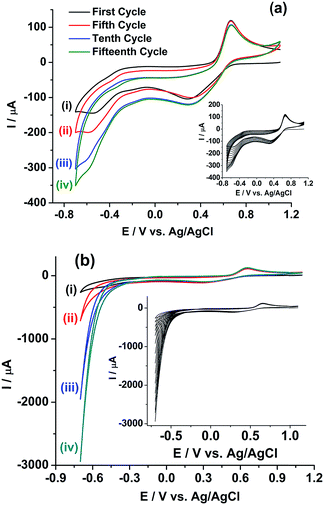Fig. 7 Cyclic voltammograms of (U, Pu)C FBTR fuel sample on (a) rGO/GC and (b) GC electrodes at a scan rate of 50 mV s−1 for (i) first, (ii) fifth, (iii) tenth and (iv) fifteenth cycle. The insets show the continuous cyclic voltammograms of 15 cycles.

Therefore, either (1) rGO/GC or (2) Pu leads to the autocatalytic reduction of U(VI) in the FBTR sample.46 Therefore, a similar experiment is performed on GC electrode. Fig. 7b shows the cyclic voltammogram of the sample on GC in the potential range of 1.1 V to −0.7 V for continuous 15 cycles at a scan rate of 50 mV s−1. The cathodic peak current of U(VI)/U(IV) reaction is similarly increased during the forward scan (i.e., scanning towards the negative potentials) in each successive cycle. This indicates that rGO/GC (or the type of the working electrode) is not responsible for the instability of the cathodic peak current of U(VI)/U(IV) in the sample solution; rather the presence of plutonium plays a crucial role in this autocatalytic reaction. That intuition is confirmed by the cyclic voltammograms of 5 mM U(VI) solution in 1 M H2SO4 on (a) rGO/GC and (b) GC electrode at the scan rate of 50 mV s−1 (Fig. 8). The cathodic peak current of U(VI)/U(IV) reaction is decreased in each successive cycle for both the electrodes. The oxidation of U(VI)/U(IV) is rather slow during the positive potential scan due to the formation of metal oxygen bond. Therefore, the decrease in the cathodic peak in each successive cycle is attributed to the decrease in the effective electrode surface area due to the deposition of insoluble uranium(IV) sulphate species on electrode.47,48 Moreover, for almost same amount of U(VI) (∼5–6 mM), the cathodic peak current of U(VI)/U(IV) reaction in pure U(VI) solution is observed as almost one third of the corresponding peak current observed in the sample solution. Therefore, in corroboration with the interfacial redox interferences of U(IV) and Pu(IV) as discussed in the introduction, it is proved that the reduction of U(VI)/U(IV) is auto-catalysed by the presence of Pu(IV) in 1 M H2SO4.49,50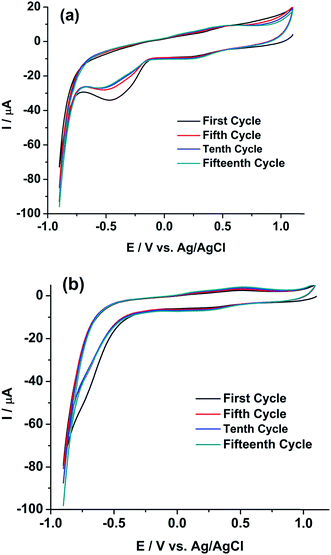Fig. 8 Cyclic voltammograms of 5 mM U(VI) in 1 M H2SO4 on (a) rGO/GC and (b) GC electrodes at a scan rate of 50 mV s−1 for (i) first, (ii) fifth, (iii) tenth and (iv) fifteenth cycle.

Fig. 9 shows a comprehensive representation of the autocatalytic reduction of U(VI) in the presence of Pu(IV) in the FBTR fuel sample solution in 1 M H2SO4. The sample solution contains uranium in U(VI) state and plutonium in Pu(IV) and Pu(VI) (to some extent) states (Zone I of the first cycle). During the potential scan towards the cathodic direction, Pu(IV) and Pu(VI) are electrochemically reduced to Pu(III) at the working electrode as shown in the Zone II. After the cathodic peak of Pu, the mass transfer of Pu(IV) and Pu(VI) towards the working electrode becomes diffusion controlled (Zone III), but U(VI) exists as it is in the solution. In Zone IV, the U(VI) starts electrochemically reduced to U(IV) and gets deposited on the working electrode as uranium(IV)–sulphate species, which comes into the direct contact of the diffusing Pu(IV) near to the working electrode. Therefore, U(VI) is regenerated near the electrode solution interface by the chemical reaction with Pu(IV) following eqn (8) and it leads to the higher reduction current of uranium.

 2[Pu(IV)]4+ + [U(IV)]4+ + 2H2O = 2[Pu(III)]3+ + [U(VI)O2]2+ + 4H+ (8)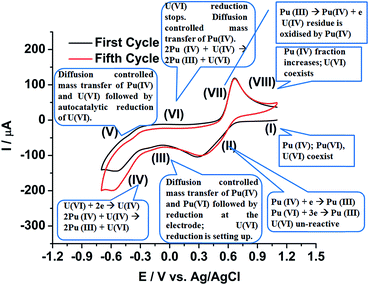Fig. 9 Comprehensive representation of the autocatalytic reduction of U(VI) in the FBTR fuel sample solution containing both uranium and plutonium in 1 M H2SO4.

The reaction (8) is very fast in H2SO4 medium.49,50 This reaction in addition to the diffusion controlled mass transfer of both U(VI) and Pu(IV) continues in the Zone V although the scan direction is reversed. The reduction of U(VI) stops in Zone VI; but the remaining U(IV) deposit, if still exists on the working electrode at all, continuously reacts with the Pu(IV) diffusing from the bulk of the solution towards the working electrode. Therefore, U(VI) accumulates near the working electrode. The Pu(III) generated till Zone VI, starts oxidising in Zone VII to Pu(IV) on the electrode. The Pu(IV) is preferentially stabilized by the supporting electrolyte (1 M H2SO4) compared to Pu(III). The diffusion controlled oxidation of Pu(III) occurs in Zone VIII. The fraction of Pu(IV) in the sample solution increases after completion of each cycle resulting into an increase in the flux of Pu(IV) from bulk of the solution to the working electrode assisting in the autocatalytic reduction of U(VI). Therefore, it is understood that unless a strategic methodology could be developed, the quantitative voltammetric measurement of U(VI) in the presence of Pu(IV) in 1 M H2SO4 is not advisable on either any simple or modified working electrode.

Therefore, we have restricted our following studies to Pu only. Fig. 10(a) shows the ASWV of the blank H2SO4 on rGO/GC electrode (i). The anodic peak of the Pu(IV)/Pu(III) redox couple of the sample is appeared at 0.541 V with the peak current of 62.14 ± 1.08 μA (ii). The anodic peak current of the same reaction is increased systematically for the addition of (iii) 1.088 mg g−1, (iv) 1.904 mg g−1, (v) 2.539 mg g−1 and (vi) 3.047 mg g−1 of standard plutonium solution into the sample. Fig. 10(b) shows the mean peak current (along with the standard deviation for ten number of repeatable measurements) of the sample at 0 value of the x-axis. The mean peak currents (along with the standard deviation for ten number of repeatable measurements) for each standard addition of plutonium is increased linearly with a sensitivity of 12.5 μA (mg g−1)−1; which is much lower compared to the sensitivity of rGO/GC observed (25.8 μA (mg g−1)−1) in pure plutonium solution. Therefore, the coexistence of uranium decreases the sensitivity of the voltammetric determination of plutonium. The sensitivity of the GC electrode for the same type of analysis is calculated as 5.35 μA (mg g−1)−1. The concentration of plutonium in the sample is evaluated as 3.222 ± 0.179 mg g−1 by extrapolating the straight line to the negative x-axis intercept (i.e. considering the y-axis value as 0) (Fig. 10(b)). The relative accuracy error (from the biamperometric result) and the relative standard deviation (of ASWV results) are calculated as −15% and 5.5%, respectively. Therefore, it is clear that there is enough scope to increase the analytical merits of the voltammetric determination of plutonium in (U, Pu)C samples.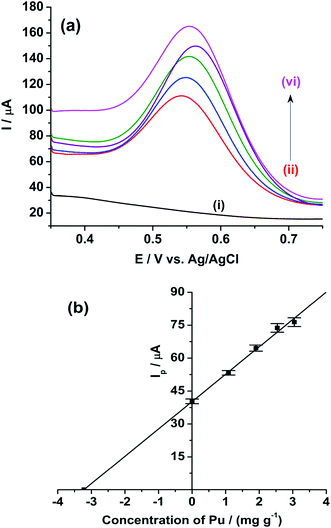Fig. 10 ASWVs of the (i) blank 1 M H2SO4 (ii) of (U, Pu)C FBTR sample in 1 M H2SO4 and standard addition of (iii) 1.088 mg g−1, (iv) 1.904 mg g−1, (v) 2.539 mg g−1 and (vi) 3.047 mg g−1 of standard plutonium solution into the sample solution. Square wave amplitude = 25 mV; square wave frequency = 20 Hz; step potential increment = 1 mV; standby time at 0.35 V is 10 s. The error bars represent the standard deviation for ten repetitive measurements.

Seven rGO/GC electrodes are prepared under the previously discussed optimized method. The mean ASWV peak currents (along with the standard deviation for ten number of repeatable measurements) of Pu(IV)/Pu(III) couple recorded with seven rGO/GCs in the final test solution (i.e.; sample + 3.047 mg g−1 of standard Pu) is shown in Fig. 11. The variation in the peak current values is attributed to the variation in the surface coverage of the rGO on the actual GC surface. It should be noted that the drop casting operation of GO on GC electrode followed by slow drying under IR lamp inside a radioactive fume hood or glove box is a tedious job justifying the variation in the relative surface coverage of rGO on GC electrode. Among the seven rGO/GCs; three electrodes (i.e. rGO4. rGO5 and rGO7) belong to the same class with 99% confidence level (0.05 < p-significance < 0.01 for F(2, 27) = 4.97). Further, the rGO/GC electrodes have showed very robust performance during the electroanalysis of actinides in acid solutions and no significant depreciation of the peak current is observed even after long operation period.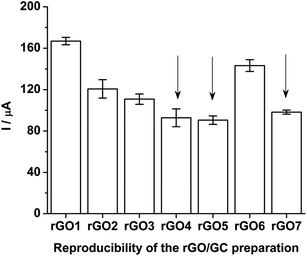Fig. 11 ASWV peak currents of Pu(IV)/Pu(III) redox couple recorded on seven rGO/GCs in the final test solution (i.e.; sample + 3.047 mg g−1 of standard Pu). The error bars represent the standard deviation for ten repetitive measurements. The arrows show the rGO/GCs selected for the ANOVA test.

## 4. Conclusions

The aqueous electrochemistry of plutonium on rGO/GC is systematically investigated for the first time in 1 M H2SO4. The increase in the extent of the reversibility as well as the enhancement of the electron transfer rate constant of Pu(IV)/Pu(III) redox reaction confirms that rGO/GC can catalyse the redox chemistry of Pu(IV)/Pu(III). For the voltammetric determination of Pu in 1 M H2SO4, the anodic voltammetric peak representing the oxidation of Pu(III) to Pu(IV) is more suitable compared to the cathodic voltammetric peak representing the reduction of Pu(IV) to Pu(III). In standard Pu solution, rGO/GC shows higher sensitivity for the determination of Pu compared to the bare GC electrode. However, the sensitivity of rGO/GC electrode decreases in the test sample solution due to the interference of uranium. Moreover, it is evidenced that the cathodic reaction of U(VI)/U(IV) redox couple is autocatalysed by the reaction with Pu(IV) diffusing from the bulk of the solution to the vicinity of the working electrode. That autocatalytic cathodic reaction of U(VI) is observed for both GC and rGO/GC electrodes. Therefore, from the reported evidences and according to our present understandings, it can be concluded that uranium cannot be determined quantitatively in the presence of plutonium on solid electrodes (irrespective of the type of surface modifications) by simple voltammetric methods. Although rGO/GC shows good analytical robustness, reproducibility, repeatability, requirement of no additional reagents as well as lesser operational duration; but the analytical merit for the determination of Pu in nuclear fuel sample is not very competitive to the biamperometric method. Therefore, a scope of research still exists for the development of simple, fast, robust, precise and accurate electroanalytical methods for the simultaneous determination of U and Pu in nuclear fuel.

## Notes and references

1. G. Srinivasan, K. V. Suresh Kumar, B. Rajendran and P. V. Ramalingam, Nucl. Eng. Des., 2006, 236, 796–811 CrossRef CAS.
2. T. Hashimoto, K. Taniguchi, H. Sugiyama and T. Sotobayashi, J. Radioanal. Chem., 1979, 52, 133–142 CrossRef CAS.
3. K. Suresh Kumar, P. Magesvaran, D. Sreejeya, T. Kumar, B. Shreekumar and P. K. Dey, J. Radioanal. Nucl. Chem., 2010, 284, 457–460 CrossRef CAS.
4. A. N. Dubey, G. R. Relan and S. Vaidyanathan, J. Radioanal. Nucl. Chem., 1999, 240, 741–746 CrossRef CAS.
5. L. Couston, J. Delage and D. Pouyat, Direct, remote, and simultaneous determination of uranium and plutonium in eight different media by optical absorptometry using nonimmersed optical fibre sensors, Proc. SPIE 3483, European Workshop on Optical Fibre Sensors, 1998 Search PubMed.
6. G. R. Relan, A. N. Dubey and S. Vaidyanathan, J. Radioanal. Nucl. Chem., 1996, 204, 15–22 CrossRef CAS.
7. C.-G. Lee, D. Suzuki, Y. Saito-Kokubu, F. Esaka, M. Magara and T. Kimura, Int. J. Mass Spectrom., 2012, 314, 57–62 CrossRef CAS.
8. R. Kapsimalis, D. Glasgow, B. Anderson and S. Landsberger, J. Radioanal. Nucl. Chem., 2013, 298, 1721–1726 CrossRef CAS.
9. N. Gopinath, G. A. Rama Rao, V. K. Manchanda and P. R. Natarajan, J. Radioanal. Nucl. Chem., 1985, 96, 273–280 CrossRef CAS.
10. A. R. Eberle, M. W. Lerner, C. G. Goldbeck and C. J. Rodden, Titrimetric determination of uranium in products, fuel and scrap materials after ferrous ion reduction in phosphoric acid, New Brunswick Laboratory, 1970 Search PubMed.
11. P. R. Nair, K. V. Lohithakshan, M. Xavier, S. G. Marathe and H. C. Jain, J. Radioanal. Nucl. Chem., 1988, 122, 19–26 CrossRef CAS.
12. G. A. Rama Rao, V. K. Manchanda and P. R. Natarajan, J. Radioanal. Nucl. Chem., 1985, 94, 95–100 CrossRef.
13. M. Xavier, P. R. Nair, K. V. Lohithakshan, S. G. Marathe and H. C. Jain, J. Radioanal. Nucl. Chem., 1991, 148, 251–256 CrossRef CAS.
14. M. V. Ramaniah, P. R. Natarajan and P. Venkataramana, Radiochim. Acta, 1975, 22, 199–213 CrossRef CAS.
15. P. R. Nair, M. Xavier and S. K. Aggarwal, Radiochim. Acta, 2009, 97, 419–422 CrossRef CAS.
16. P. H. Chadwick and I. R. McGowan, Talanta, 1972, 19, 1335–1348 CrossRef CAS PubMed.
17. W. Davies and W. Gray, Talanta, 1964, 11, 1203–1211 CrossRef CAS.
18. J. L. Drummond and R. A. Grant, Talanta, 1966, 13, 477–488 CrossRef CAS PubMed.
19. W. D. Shults, Talanta, 1963, 10, 833–849 CrossRef.
20. G. W. C. Milner, G. Phillips and A. J. Fudge, Talanta, 1968, 15, 1241–1257 CrossRef CAS PubMed.
21. J. E. Harrar, Electroanalytical chemistry, Marcel Dekker, New York, 1975 Search PubMed.
22. T. Tanaka, G. Marinenko and W. F. Koch, Talanta, 1985, 32, 525–530 CrossRef CAS PubMed.
23. H. S. Sharma, N. B. Khedekar, S. G. Marathe and H. C. Jain, Nucl. Technol., 1990, 89, 399–405 CAS.
24. H. S. Sharma, V. Jisha, D. M. Noranha, M. K. Sharma and S. K. Aggarwal, Performance evaluation of indigenous controlled potential coulometer for the determination of uranium and plutonium, 2007, BARC Report, BARC/2007/E/012 Search PubMed.
25. M. K. Sharma, J. V. Kamat, A. S. Ambolikar, J. S. Pillai and S. K. Aggarwal, Coulometry for the determination of uranium and plutonium: Past and present 2012, BARC Report, BARC/2012/E/001 Search PubMed.
26. N. Gopinath, N. N. Mirashi, K. Chander and S. K. Aggarwal, J. Appl. Electrochem., 2004, 34, 617–622 CrossRef CAS.
27. H. S. Sharma, R. B. Manolkar, J. V. Kamat and S. G. Marathe, Fresenius. J. Anal. Chem., 1993, 347, 486–490 CrossRef CAS.
28. A. R. Joshi and U. M. Kasar, J. Radioanal. Nucl. Chem., 1991, 150, 483–491 CrossRef CAS.
29. S. K. Guin, J. S. Pillai, A. S. Ambolikar, A. Saha and S. K. Aggarwal, RSC Adv., 2013, 3, 17977–17988 RSC.
30. S. K. Guin, A. S. Ambolikar and J. V. Kamat, Electrochim. Acta, 2015, 174, 1002–1008 CrossRef.
31. R. Gupta, K. Jayachandran and S. K. Aggarwal, RSC Adv., 2013, 3, 13491–13496 RSC.
32. R. Gupta, S. K. Guin and S. K. Aggarwal, RSC Adv., 2012, 2, 1810–1819 RSC.
33. K. D. Singh Mudher and K. Krishnan, J. Alloys Compd., 2000, 313, 65–68 CrossRef.
34. K. Chander, B. N. Patil, J. V. Kamat, N. B. Khedekar, R. B. Manolkar and S. G. Marathe, Nucl. Technol., 1987, 78, 69–74 Search PubMed.
35. A. J. Bard and L. R. Faulkner, Electrochemical Methods: Fundamentals and Applications, Wiley, second edn, 2000 Search PubMed.
36. R. S. Nicholson and I. Shain, Anal. Chem., 1965, 37, 178–190 CrossRef.
37. R. S. Nicholson and I. Shain, Anal. Chem., 1965, 37, 190–195 CrossRef.
38. R. S. Nicholson, Anal. Chem., 1965, 37, 667–671 CrossRef.
39. R. S. Nicholson, Anal. Chem., 1965, 37, 1351–1355 CrossRef.
40. R. S. Nicholson and I. Shain, Anal. Chem., 1964, 36, 706–723 CrossRef.
41. J. Heinze, Angew. Chem., Int. Ed. Engl., 1984, 23, 831–847 CrossRef.
42. H. Matsuda and Y. Ayabe, Z. Elektrochem., 1955, 59, 494–503 Search PubMed.
43. X. Liu, Y. Wen, B. Shan, K. Cho, Z. Chen and R. Chen, Appl. Phys. A, 2015, 118, 885–892 CrossRef.
44. C. Gómez-Navarro, J. C. Meyer, R. S. Sundaram, A. Chuvilin, S. Kurasch, M. Burghard, K. Kern and U. Kaiser, Nano Lett., 2010, 10, 1144–1148 CrossRef PubMed.
45. A. W. Bott, Curr. Sep., 1999, 18, 9–16 Search PubMed.
46. D. Gil, R. Malmbeck, J. Spino, T. Fanghänel and E. Dinnebier Robert, Radiochim. Acta, 2010, 98, 77–89 CrossRef.
47. S. Suzuki, S. Hirono, Y. Awakura and H. Majima, Metall. Trans. B, 1990, 21, 839–844 CrossRef.
48. P. Biddle, J. H. Miles and M. J. Waterman, J. Inorg. Nucl. Chem., 1966, 28, 1736–1739 CrossRef.
49. T. W. Newton, J. Phys. Chem., 1959, 63, 1493–1497 CrossRef.
 This journal is © The Royal Society of Chemistry 2015